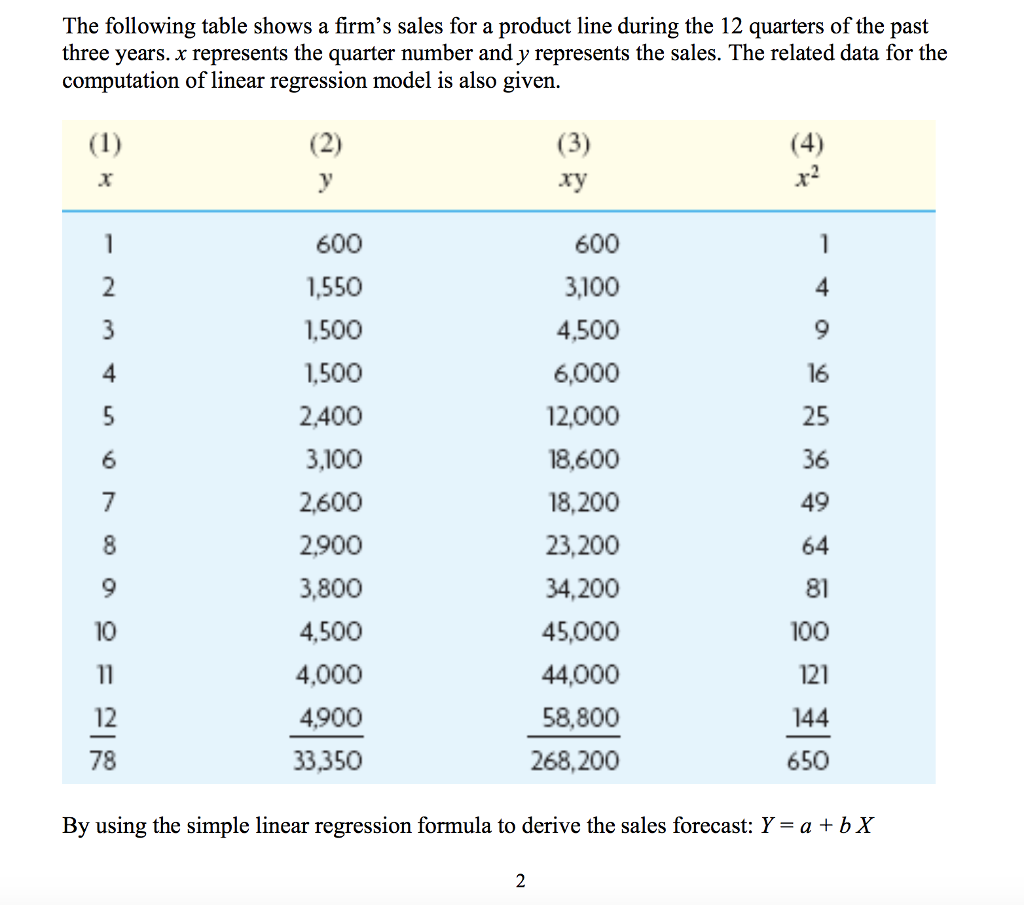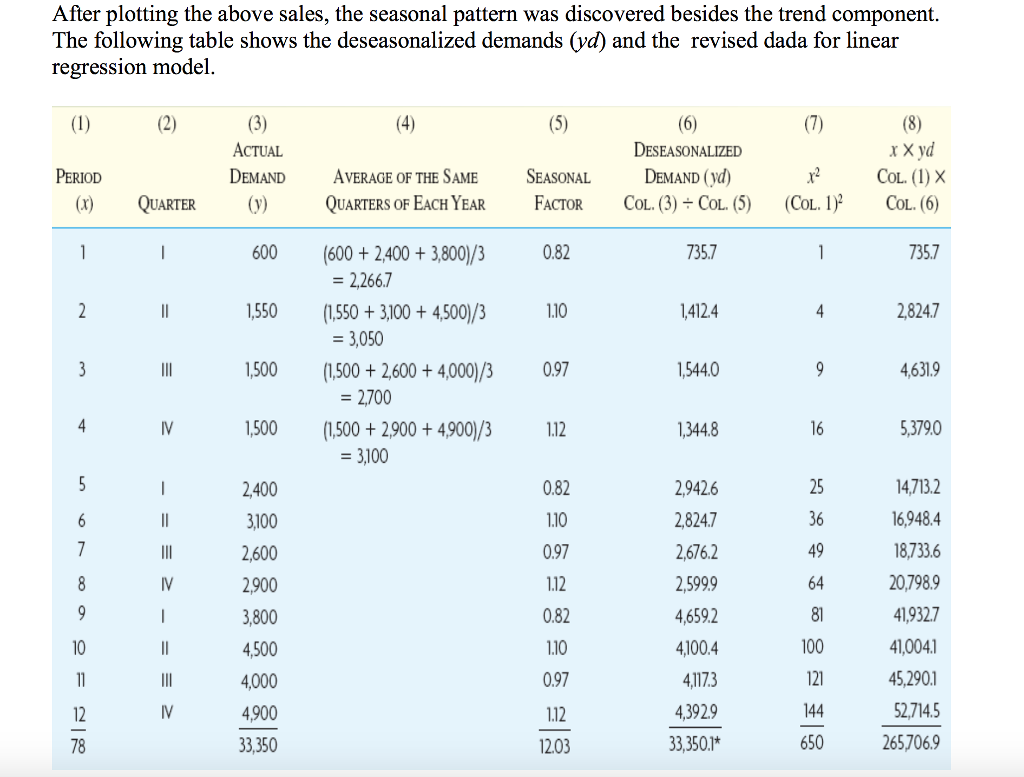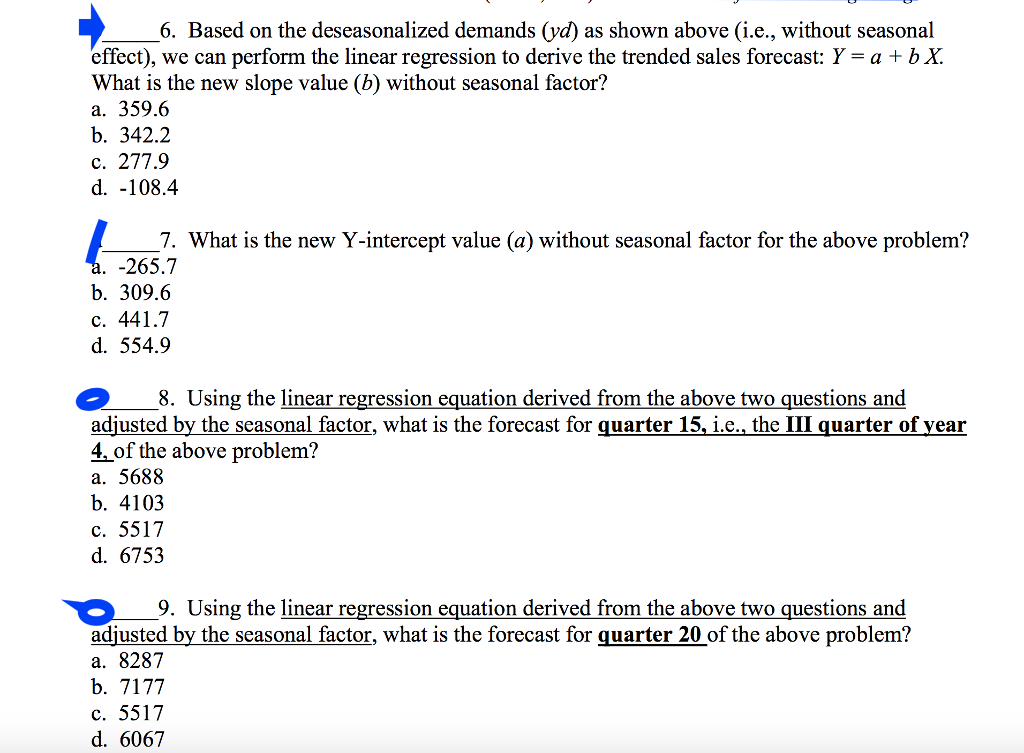# Answered! Hi Folks, I am really getting confused here, I dont know how to solve these problems based on the deseasonalized…

Hi Folks, I am really getting confused here, I dont know how to solve these problems based on the deseasonalized demands (yd) (i.e., without seasonal effect) by using simple linear regression formula to derive the sales forecast: Y = a + b X . Please help me to find the slope value (b) and Y- intercept value (a) and the forcast for quater 15, i.e., the III quater of year 4, and the forcast for quater 20 of the above problem . I would really apprecite it. Please please show me the work and how you came to the answers? I dont need directly answers without showing me the calcualtions and the method how you got to those answers please. I want to learn and understand the materials.In a Linear Regression Equation , where:

Y = a + b.x

Don't use plagiarized sources. Get Your Custom Essay on
Answered! Hi Folks, I am really getting confused here, I dont know how to solve these problems based on the deseasonalized…
GET AN ESSAY WRITTEN FOR YOU FROM AS LOW AS \$13/PAGE

( Sum of all y values)x (Sum of all x^2 values) – (sum of all x values)x(sum of all xy values)

A = ——————————————————————————————————————-

n x ( Sum of all x^2 values) – ( sum of x values)^2

n x (sum of all values which are product of x.y) – ( sum of x values)x(sum of y values)

B = ———————————————————————————————————————-

n x ( Sum of all x^2 values) – ( sum of x values)^2

Here,

A = Y intercept value

B = Slope Value

N = number of data = 12

Sum of x values = 78

Sum of y values ( deseasonalized demand) = 33350.1

Sum of all values which are product of x.y = 265706.9

Sum of all x^2 values = 650

Therefore ,

33350.1 x 650 – 78 x 265706.9          21677565 – 20725138.2                952426.8

A=          —————————————   =      ——————————     =      ————– =   555.02

12 x 650 – 78×78                                   7800 – 6084                                   1716

12 X 265706.9 – 78 X 33350.1     3188482.8   – 2601307.8           587175

B =          ———————————–   =         —————————   =    ————-   =      342.17

12 X 650 – 78 X 78                             7800 – 6084                             1716

( OR 342.2 ROUNDED TO ONE DECIMAL POINT)

Therefore Linear regression Equation is :

Y =   555.02 + 342.17.X

Y = Forecast value for sales

X = Quarter number

Thus in order to find out sales value for quarter 16, we need to find out value of Y for X = 15

Accordingly,

Y = 555.02 + 342.17 x 15 = 555.02 + 5132.55 = 5687.57 ( 5688 rounded to nearest whole number)

Note : Value of Y 555.02 is nearest to value 554.9 ( which is one of the options in answer to question 7). Therefore answer to question 7 will be “554.9”

Similarly Forecast for quarter 20 will be:

Y = 555.02 + 342.17 X 20 = 555.02 + 6843.4 = 7398.42